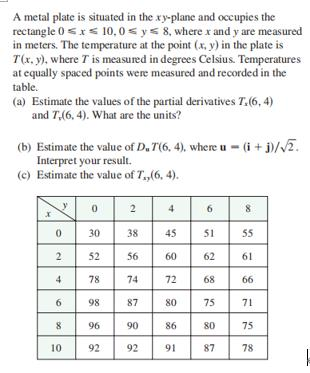### ¿Todavía tienes preguntas de matemáticas?

Pregunte a nuestros tutores expertos
Algebra
PreguntaA metal plate is situated in the $$x y$$ -plane and occupies the rectangle $$0 \leq x \leq 10,0 \leq y \leq 8$$ , where $$x$$ and $$y$$ are measured in meters. The temperature at the point $$( x , y )$$ in the plate is $$T ( x , y )$$ . where $$T$$ is measured in degrees Celsius. Temperatures at equally spaced points were measured and recorded in the table.

(a) Estimate the values of the partial derivatives $$T _ { x } ( 6,4 )$$ and $$T _ { v } ( 6,4 )$$ . What are the units?

(b) Estimate the value of $$D _ { u } T ( 6,4 )$$ , where $$u = ( i + j ) / \sqrt { 2 }$$ . Interpret your result.

(c) Estimate the value of $$T _ { x p } ( 6,4 )$$

$$\left. \begin{array} { l } { \text { 11. (a) } \approx 3.5 ^ { \circ } C / m , - 3.0 ^ { \circ } C / m } \\ { \text { (b) } \approx 0.35 ^ { \circ } C / m \text { by Equation } 14.6 .9 \text { (Definition } 14.6 .2 \text { gives } } \\ { \approx 1.1 ^ { \circ } C / m . ) } \\ { \text { (c) } - 0.25 } \end{array} \right.$$